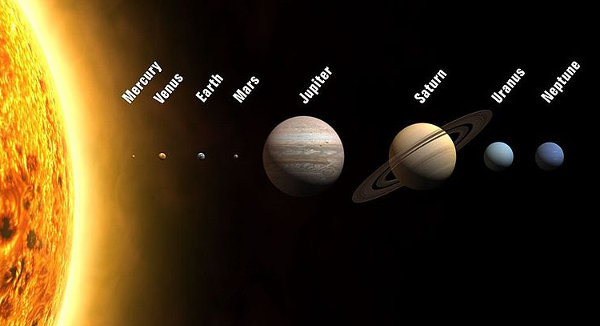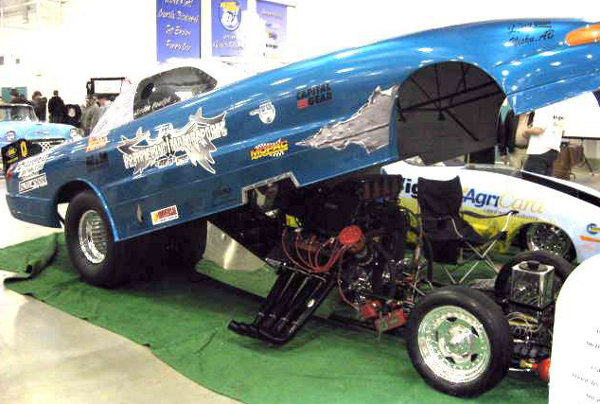# Ratio

A ratio is a relationship between two quantities of the same type.

For example, 2:1 may represent the ratio of girls to boys in a class. This means that there are 2 times as many girls as there are boys in the class.

Ratios are commonly used in:

• House plans - A scale of 1:100 means that the real house is 100 times larger than the measurements shown on the plan.
• Maps - A scale of a map of China may be 1:10000. This means that the real distance is 10 000 times the distance measured on the map.
• Design drawings of small objects - To make small watch parts, designs may have scales of 20:1 where the real watch part is 120 of the length shown on the design diagram.
• Two-stroke engine fuel ratio - In motorbikes and lawnmowers with two-stroke engines, the fuel components of gasoline and oil are mixed in the ratio of 50:1.

## Maths Fun - Waist to Hip Ratio

The Waist-Hip Ratio is used by doctors to determine a person's "fatness", a risk factor in cardio-vascular (heart) disease. For adult women, a WHR less than 8.5 is healthy. For adult men, a WHR less than 9 is healthy.

Use a tape measure to measure around your waist and your hips. Divide your waist measurement by your hip measurement. How did you do?

## Example One - Simplifying Ratios

Simplify these ratios by dividing both numbers by the greatest common factor.

10 : 2 = 5 : 1

3 : 6 = 1 : 2

25 : 30 = 5 : 6

\$4 : 60 cents = 400 cents : 60 cents = 400 : 60 = 20 : 3

5 kilometres:200 metres = 5000 metres:200 metres = 25:1

## Questions

Simplify these ratios:
Q1. 30 : 9
Q2. 45 : 18
Q3. \$4.20 : 14 cents

A1. 10 : 3
A2. 5 : 2
A3. 30 : 1

## Example Two - Solar System to ScalePLANET AVERAGE DISTANCE FROM SUN (KM) Mercury 57 million Venus 108 million Earth 150 million Mars 228 million Jupiter 779 million Saturn 1 430 million Uranus 2 880 million Neptune 4 500 million

My homework assignment is to draw a line marking the Sun and then all 8 planets' positions. The distances must be to scale. I only have A4 photocopy paper which is 30 cm long. What scale should I use? What will be the scaled planetary distances?

Estimated scale = 4 500 000 000 ÷ 30 = 150 000 000
If I use this scale, I won't be able to fit the names of the Sun and Neptune on my paper so I need a larger scale. I'll use a scale of 1: 200 000 000.

Now, I'll divide all the real distances by 200 000 000 e.g.
Scaled distance of Neptune = 4 500 000 000 ÷ 200 000 000 = 22.5 cm

 PLANET SCALED AVERAGE DISTANCE FROM SUN Mercury 0.285 cm or 2.85 mm Venus 0.54 cm or 5.4 mm Earth 0.75 cm or 7.5 mm Mars 1.14 cm Jupiter 3.895 cm Saturn 7.15 cm Uranus 14.4 cm Neptune 22.5 cm

## Questions - Scale Drawings

A house plan has a scale of 200:1 which means that the real house is 200 times larger than the measurement shown on the plan. Because carpenters must work accurately, house plan dimensions are marked in millimetres. Change these room dimensions to show the lengths that would be drawn on a house plan.

Q1. Bedroom - 3000 mm by 4000 mm
Q2. Real Bathroom - 2500 mm by 2400 mm

A1. Bedroom - 15 mm by 20 mm
A2. Bathroom - 12.5 mm by 12 mm

## Did You Know That...?

A remote sensing system called Geographic Information System (GIS) is used to map locations for:

• identifying geographic features such as mountains, rivers and coastlines
• identify areas suitable for mining ores such as iron which is magnetic
• detecting environmental contamination such as oil spills
• locating underground water aquifers
• locating underground archaeological sites.

## Example Three - Drag Car FuelDrag car fuel is a mixture in the ratio of 9:1 (9 parts Nitromethane and 1 part Methanol). A drag car can consume up to 120 litres of fuel for one quarter-mile sprint including the warm-up. How much of this is Nitromethane and how much is Methanol?

Total number of parts = 9 + 1 = 10 parts

Quantity of Nitromethane = 910 × 120 = 108 litres

Quantity of Methanol = 110 × 120 = 12 litres

## Question - Concrete

Concrete is made in the ratio of 1:2:3. This means 1 part of cement, 2 parts of sand and 3 parts of gravel. How much of each would I need to make a concrete house slab with a volume of 90 cubic metres?